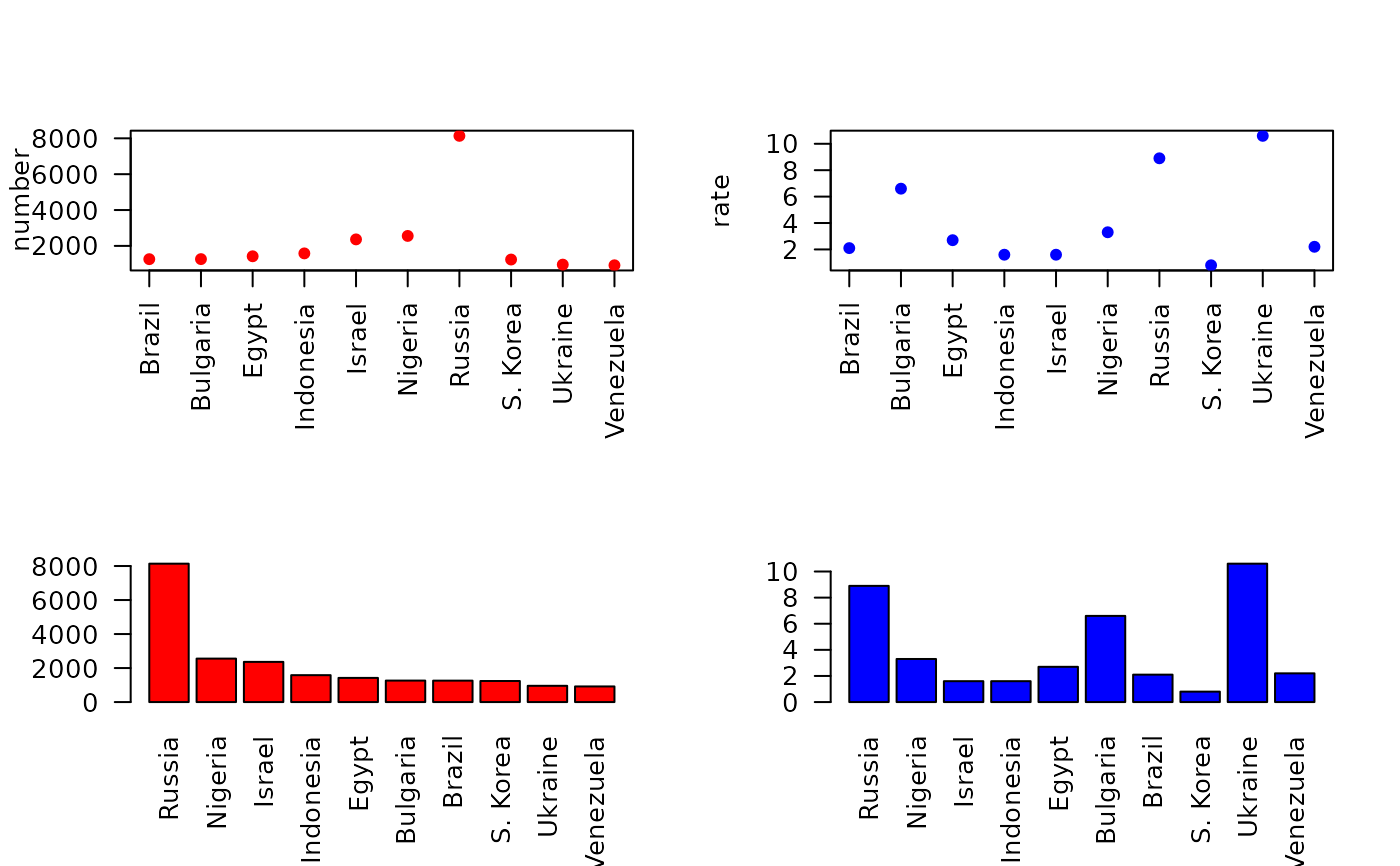Data for Exercises 1.14 and 1.37

Diplomat

## Format

A data frame/tibble with 10 observations on three variables

country

a factor with levels Brazil, Bulgaria, Egypt, Indonesia, Israel, Nigeria, Russia, S. Korea, Ukraine, and Venezuela

number

total number of tickets

rate

number of tickets per vehicle per month

## Source

Time, November 8, 1993. Figures are from January to June 1993.

## Examples


par(las = 2, mfrow = c(2, 2))
stripchart(number ~ country, data = Diplomat, pch = 19,
col= "red", vertical = TRUE)
stripchart(rate ~ country, data = Diplomat, pch = 19,
col= "blue", vertical = TRUE)
with(data = Diplomat,
barplot(number, names.arg = country, col = "red"))
with(data = Diplomat,
barplot(rate, names.arg = country, col = "blue"))par(las = 1, mfrow = c(1, 1))
if (FALSE) {
library(ggplot2)
ggplot2::ggplot(data = Diplomat, aes(x = reorder(country, number),
y = number)) +
geom_bar(stat = "identity", fill = "pink", color = "black") +
theme_bw() + labs(x = "", y = "Total Number of Tickets")
ggplot2::ggplot(data = Diplomat, aes(x = reorder(country, rate),
y = rate)) +
geom_bar(stat = "identity", fill = "pink", color = "black") +
theme_bw() + labs(x = "", y = "Tickets per vehicle per month")
}